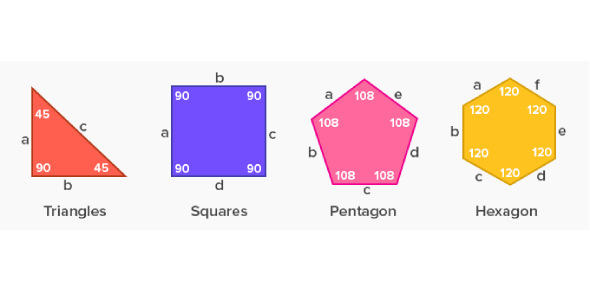Take This Important Geometry Trivia Test!

18 Questions | Total Attempts: 420SettingsIf you are having a hard time in geometry class and are looking for a way to refresh your understanding on the topic, you have come to the right place as this important quiz will do just that for you. It has some of the topics that most students have a hard time answering. Feel free to take this as much as you can.

• 1.
Which best describes the statement If two planes intersect, then their intersection is a point?
• A.

Always

• B.

Sometimes

• C.

Never

• D.

Cannot tell

• 2.
Choose the property that justifies the following statement.If x = 2 and x + y = 3, then 2 + y = 3.
• A.

Reflexive

• B.

Symmetric

• C.

Transitive

• D.

Substitution

• 3.
Choose the property that justifies the statement mA = mA.
• A.

Reflexive

• B.

Symmetric

• C.

Transitive

• D.

Substitution

• 4.
On a line, if XY = 6, YZ = 4, and XZ = 2, which point is between the other two?
• A.

X

• B.

Y

• C.

Z

• D.

Cannot tell

• 5.
If mBFC = 70, find mEFD.
• A.

10

• B.

20

• C.

35

• D.

70

• 6.
If mAFB = 5x – 10 and mBFC = 3x + 20, find x.
• A.

10

• B.

15

• C.

21.25

• D.

23.33

• 7.
If ∠ABC ≅ ∠EFG, and mABC = 72, find mGFH.
• A.

18

• B.

72

• C.

90

• D.

108

• 8.
If mABJ = 28, ∠ABC ≅ ∠DBJ, find mJBC.
• A.

90

• B.

56

• C.

45

• D.

34

• 9.
If m∠1 = x + 50 and m∠2 = 3x – 20, find m∠1.
• 10.
State the definition, property, postulate, or theorem that justifies each statement.If ∠A ≅ ∠B and ∠B ≅ ∠C, then ∠A ≅ ∠C.
• 11.
State the definition, property, postulate, or theorem that justifies each statement.If mA + mB = 90 and mB = 20, then mA + 20 = 90.
• 12.
State the definition, property, postulate, or theorem that justifies each statement.If ∠X and ∠Y are complementary, ∠Z and ∠Q are complementary, and ∠X ≅ ∠Z, then ∠Y ≅ ∠Q
• 13.
State the definition, property, postulate, or theorem that justifies each statement.AB + BC = AC
Related TopicsBack to top